Next: Angular momentum Up: Rotational motion Previous: Worked example 8.6: Horsepower

## Worked example 8.7: Rotating cylinder

Question: A uniform cylinder of radius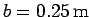is given an angular speed of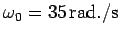about an axis, parallel to its length, which passes through its centre. The cylinder is gently lowered onto a horizontal frictional surface, and released. The coefficient of friction of the surface is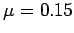. How long does it take before the cylinder starts to roll without slipping? What distance does the cylinder travel between its release point and the point at which it commences to roll without slipping?Answer: Letbe the velocity of the cylinder's centre of mass,the cylinder's angular velocity,the frictional force exerted by the surface on the cylinder,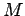the cylinder's mass, and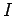the cylinder's moment of inertia. The cylinder's translational equation of motion is written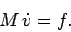Note that the friction force acts to accelerate the cylinder's translational motion. Likewise, the cylinder's rotational equation of motion takes the form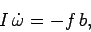since the perpendicular distance between the line of action ofand the axis of rotation is the radius,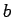, of the cylinder. Note that the friction force acts to decelerate the cylinder's rotational motion. If the cylinder is slipping with respect to the surface, then the friction force,, is equal to the coefficient of friction,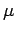, times the normal reaction,, at the surface:Finally, the moment of inertia of the cylinder isThe above equations can be solved to give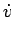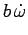Given that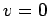(i.e., the cylinder is initially at rest) and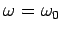at time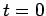, the above expressions can be integrated to give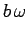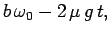which yieldsNow, the cylinder stops slipping as soon as the no slip'' condition,is satisfied. This occurs whenWhilst it is slipping, the cylinder travels a distanceNext: Angular momentum Up: Rotational motion Previous: Worked example 8.6: Horsepower
Richard Fitzpatrick 2006-02-02